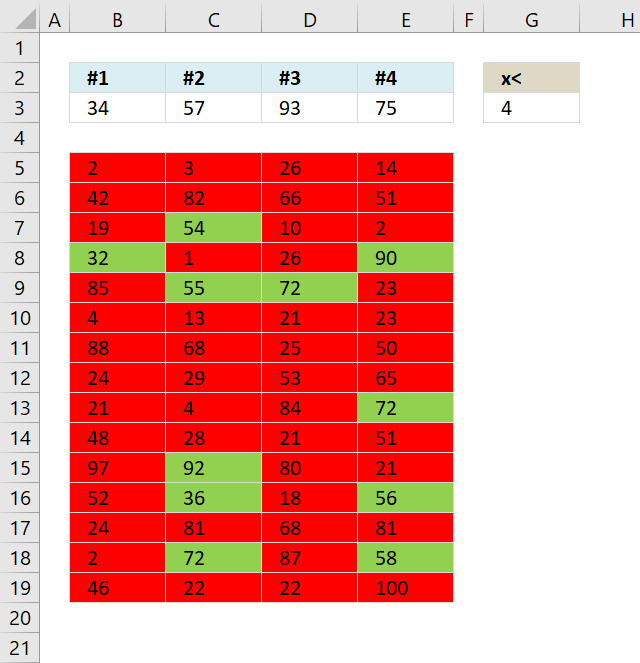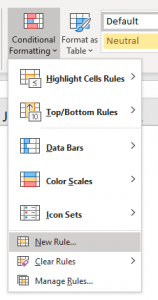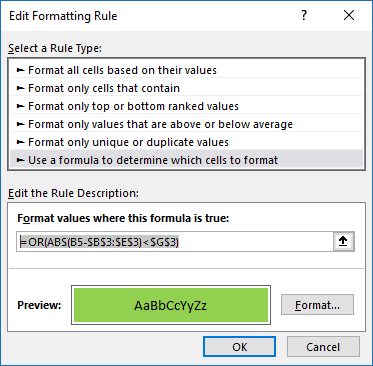Author: Oscar Cronquist Article last updated on August 29, 2019This article demonstrates how to apply Conditional Formatting formula to a cell range, it finds cells that are in close proximity to a given set of numbers based on a range value.

The image above shows 4 values in cell range B3:E3, the range number is in cell G3. A conditional formatting formula highlights cells in cell range B5:E19 that contain a number that is near at least one of the four values in cell range B3:E3.

For example, the image above shows 34, 57, 93 and 75. The range is 4. If 2 which is the cell value in B5 is in proximity with 34, 57 , 93 or 75 then cell B5 is highlighted green.

Number 34 has a higher bound 34 +4 = 38 and a lower bound of 34 - 4 = 30. 2 is not between 30 and 38.

Number 57 has a higher bound 57 + 4 = 61 and a lower bound of 57 - 4 = 53. 2 is not between 53 and 61.

Number 93 has a higher bound 93+ 4 = 97 and a lower bound of 93 - 4 = 89. 2 is not between 89 and 97.

Number 75 has a higher bound 75+ 4 = 79 and a lower bound of 75 - 4 = 71. 2 is not between 71 and 79. Cell B5 is highlighted red.

This article is inspired by a question found here.

Merit asks:

I have a large array of numbers and a row of numbers. I want each number in the array to become highlighted one color if it is within 4 of any number in the row and another if it is not.
Is this possible?Something like Abs(any number in array-any number in row < 4, Highlight green, highlight red) I think I have to utilize index or match functions but I'm having difficulty.

### Conditional formatting formulas

Green:

=OR(ABS(B5-\$B\$3:\$E\$3)<\$G\$3)

Red:

=AND(ABS(B5-\$B\$3:\$E\$3)>=\$G\$3)

You can also use this formula for highlighting cells red.

=OR(ABS(B5-\$B\$3:\$E\$3)<\$G\$3)=FALSE

### How to apply conditional formatting formulas

1. Select cell range (B5:E19)
2. Go to tab "Home" on the ribbon.
3. Click on the "Conditional formatting" button.
4. Click "New Rule...".5. Select "Use a formula to determine which cells to format".
6. Paste =OR(ABS(B5-\$B\$3:\$E\$3)<\$G\$3) to Format values where this is TRUE:7. Click "Format" button
8. Go to tab "Fill"
9. Select a color
10. Click ok
11. click ok
Repeat step 4 to 11 with "red" conditional formatting formula.

### Explaining "green" formula in cell A4

#### Step 1 - Subtract value in cell B5 with array of values specified in \$B\$3:\$E\$3

B5-\$B\$3:\$E\$3

becomes

2-{34, 57, 93, 75}

and returns

{-32, -55, -91, -73}

#### Step 2 - Return the absolute value of a number

The ABS function removes the minus sign from a number.

ABS(B5-\$B\$3:\$E\$3)

becomes

ABS({-32, -55, -91, -73})

and returns

{32, 55, 91, 73}

#### Step 3 - Check if values are less than value in cell G3

The less than character < lets you check if a number is less than another number.

ABS(B5-\$B\$3:\$E\$3)<\$G\$3

becomes

{32, 55, 91, 73}<4

and returns

{FALSE, FALSE, FALSE, FALSE}

This means that the value in cell B5 is not in proximity within any of the numbers in cell range B3:E3.

#### Step 4 - Return FALSE only if all values are FALSE

The OR function returns TRUE if at least one of the boolean values in the array is TRUE and FALSE if all values are FALSE.

OR(ABS(A4-\$A\$2:\$D\$2)<\$F\$1)

becomes

OR({FALSE, FALSE, FALSE, FALSE})

and returns FALSE. Cell B5 is not highlighted green.

### Download Excel fileMerit2-1.xlsx